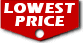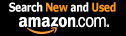# Greek Mathematical Works: Volume I, Thales to Euclid. (Loeb Classical Library No. 335) / Ivor Thomas,

List Price: \$29
Our Price: \$29For Bulk orders
Quantity

Used Book Price:
/ Ivor Thomas,
Publisher: Harvard University Press
Availability:
Sales Rank: 923326
 ISBN-10: 0674993691ISBN-13: 9780674993693

The wonderful achievement of Greek mathematics is here illustrated in two volumes of selected mathematical works. Volume I contains: The divisions of mathematics; mathematics in Greek education; calculation; arithmetical notation and operations, including square root and cube root; Pythagorean arithmetic, including properties of numbers; square root of 2; proportion and means; algebraic equations; Proclus; Thales; Pythagorean geometry; Democritus; Hippocrates of Chios; duplicating the cube and squaring the circle; trisecting angles; Theaetetus; Plato; Eudoxus of Cnidus (pyramid, cone, etc.); Aristotle (the infinite, the lever); Euclid.

Volume II (Loeb Classical Library no. 362) contains: Aristarchus (distances of sun and moon); Archimedes (cylinder, sphere, cubic equations; conoids; spheroids; spiral; expression of large numbers; mechanics; hydrostatics); Eratosthenes (measurement of the earth); Apollonius (conic sections and other works); later development of geometry; trigonometry (including Ptolemy's table of sines); mensuration: Heron of Alexandria; algebra: Diophantus (determinate and indeterminate equations); the revival of geometry: Pappus.

Now you can buy Books online in USA,UK, India and more than 100 countries.
*Terms and Conditions apply
Disclaimer: All product data on this page belongs to.
No guarantees are made as to accuracy of prices and information.